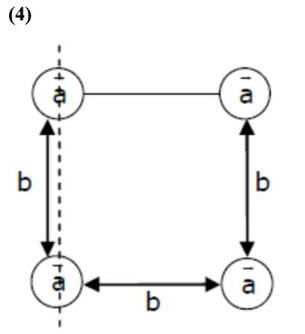# Four identical solid spheres each of mass ' m ' and radius ' a '

Question:

Four identical solid spheres each of mass ' $m$ ' and radius ' $a$ ' are placed with their centres on the four corners of a square of side ' $b$ '. The moment of inertia of the system about one side of square where the axis of rotation is parallel to the plane of the square is :

1. $\frac{4}{5} m a^{2}$

2. $\frac{8}{5} m a^{2}+m b^{2}$

3. $\frac{4}{5} m a^{2}+2 m b^{2}$

4. $\frac{8}{5} m a^{2}+2 m b^{2}$

Correct Option: , 4

Solution:$I=\frac{2}{5} m a^{2}+\frac{2}{5} m a^{2}+\left[\frac{2}{5} m a^{2}+m b^{2}\right]+\frac{2}{5} m a^{2}+m b^{2}$

$I=4 \times \frac{2}{5} m a^{2}+2 m b^{2}$

$=\frac{8}{5} m a^{2}+2 m b^{2}$